# Pie division

5/8 of a pie divide 6 pieces. Each friend got 1/6.  What fraction  of the whole pie does each person receive?

x =  0.1042

### Step-by-step explanation:

$x=\frac{5}{8}\mathrm{/}6=\frac{5}{48}=0.1042$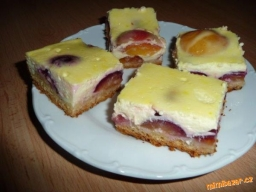Did you find an error or inaccuracy? Feel free to write us. Thank you!Tips to related online calculators
Need help to calculate sum, simplify or multiply fractions? Try our fraction calculator.

## Related math problems and questions:

• Pie II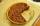Vili ate three pieces of pie. If it pieces is 1/8 how much pie did he eat?
• Strawberry-rhubarb pie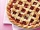Larry had 3/7 of a strawberry-rhubarb pie left over. He split the leftover pie evenly between his 3 children. What fraction of a pie did each child get?
• A baker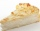A baker has 5 1/4 pies in her shop. She cut the pies in pieces that are each 1/8 of a whole pie. How many pieces of pie does she have?
• One fourth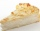One fourth of an apple pie is left for 2 family members to share equally. What fraction of the original pie will each member​ get?
• Max has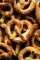Max has a 1/3 of a pound of pretzels and wants to share equal parts between himself and a friend. How much pretzels will Max's friend receive?
• Divide 13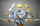Divide. Simplify your answer and write as an improper fraction or whole number. 14÷8/3
• JerryJerry has 3/4 of a pizza. He needs to share it with 6 friends. What fraction of the pizza will each friend get? Only write the fraction
• Barbara 2Barbara get 6 pizzas to divide equally among 4 people. How much of a pizza can each person have?
• One half 2One half pizza will be divide among 3 pupils. Each pupil receive 1/6. Is it true or false?
• Candy barsSheldon has 4 candy bars and wants to split them among his five friends. If each person gets the same amount, what part of the candy bar will each friend get? Show your work.
• Divide 11Divide the product of 4 and 5/8 by 1 1/2. Write your answer as a mixed number.
• Division by zero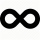Fraction 5 by 2. if 3 is added to numerator and 2 is subtracted from the denominator then the new fraction is:
• Product of two fractionsProduct of two fractions is 9 3/5 . If one of the fraction is 9 3/7. Find the other fraction.
• What fraction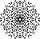What fraction of number 1 to 30 is prime?
• Kate shares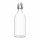Kate shares a 64-ounce bottle of apple cider with five friends. Each person's serving will be the same number of ounces. Between what two whole number of ounces will each person's serving to be? Explain using division.
• Fraction unknowns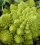Divide of fractions with unknowns: Fraction 1: The quantity x squared plus 6 times x plus 9 over the quantity x minus 1. Fraction 2: the quantity x squared minus 9 over the quantity x squared minus 2 times x plus 1.  Find Fraction 1 over Fraction 2.
• Pumpkin pie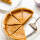Have some pumpkin pie. One half of the pie is cut into 4 equal slices, the other half is cut into 3 equal slices. After eating one of the larger slices (on the 3 piece side), I wonder if I will eat more if I have one more from the 3 side, or two from the